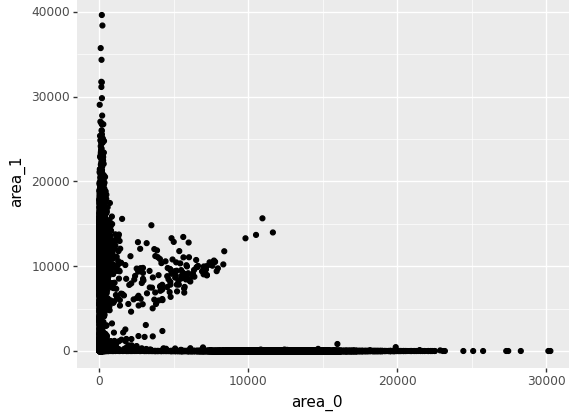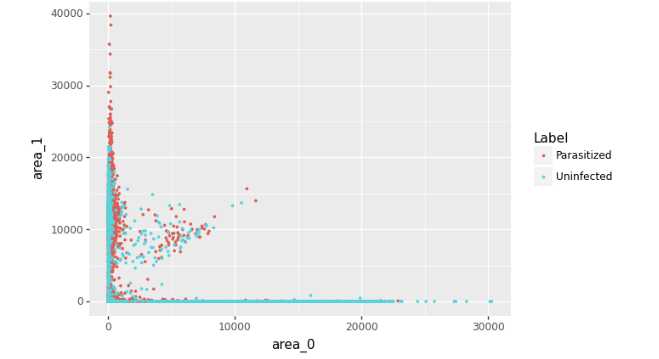An Introduction to Grammar of Graphics for Python

• Last Updated : 16 Jul, 2021

A grammar of graphics is basically a tool that enables us to describe the components of a given graphic. Basically, what this allows us to see beyond the named graphics, (scatter plot, to name one) and to basically see the underlying statistics behind it. The grammar of graphics was originally introduced by Leland Wilkinson in the 1990s and was popularized by Hadley Wickham with ggplot.

Components of Grammar of graphics

Typically, to build or describe any visualization with one or more dimensions, we can use the components as follows.

• Data
Data is an essential component of graphical grammar. After all, it contains all the information that we need to visualize. Therefore, it is important to know what is the format of the data, and what information we are working with.
• Layer
Basically, a layer is something that you can relate to in real life as well. We can think of layers as a transparent sheet containing a graphic, which can be arranged and combined in a variety of ways.
• Geom
The visual display of geom is known as geom. A geom could be a line, point, or even a bar, pie, etc. We can display a lot of information by “layering” geoms.
• Scaling data
It is very useful to re-scale our data. Scaling data does not change the data, as per say, it just changes the viewpoint of the dataset.

This grammar of graphics was first introduced in R, using ggplot and ggplot2. Considering its success in the past, it is also been introduced in Python as plotnine.

Python binding

plotnine is an implementation/binding of a grammar of graphics in Python. It is based on ggplot2. So, basically, if you’re familiar with R programming and ggplot2, chances are that you would catch up with plotnine in almost no time. There are only 2 noticeable changes in ggplot2 and plotnine.

• In R, a plus sign indicates the code/instruction to continue to the next line. However, if we do the same thing in python, it throws an exception. To cover this, in plotnine, the expression before the plus sign is enclosed in braces and so, could be used like that.
• The column name must be strings. This is more likely a feature of R, where you could pass the column name as a function argument without enclosing it in quotes. However, in Python, if the word is not enclosed in double quotes or single quotes, it would treat the word as a variable.

Installation

This module does not come built-in with Python. To install this module type the below command in the terminal.

pip install plotnine

Note: Here’s the link to the CSV file used in the below examples:dataset.csv

Example 1:

Python3

 import pandas as pdfrom plotnine import *  # load datasetdataset = pd.read_csv("dataset.csv") # ggplot is to plot the given data(ggplot(dataset, aes(x = "area_0", y = "area_1"))+    geom_point()) # aes contains parameters which work# as x-axis and y-axis for the given plot# geom.point() makes the data entries as points

Output:Example 2:

Python3

 import pandas as pdfrom plotnine import *  # load datasetdataset = pd.read_csv("dataset.csv") (ggplot(dataset, aes(x = "area_0", y = "area_1"))+    geom_point(color = "label", alpha = 0.7,               size = 0.5))

Output:My Personal Notes arrow_drop_up